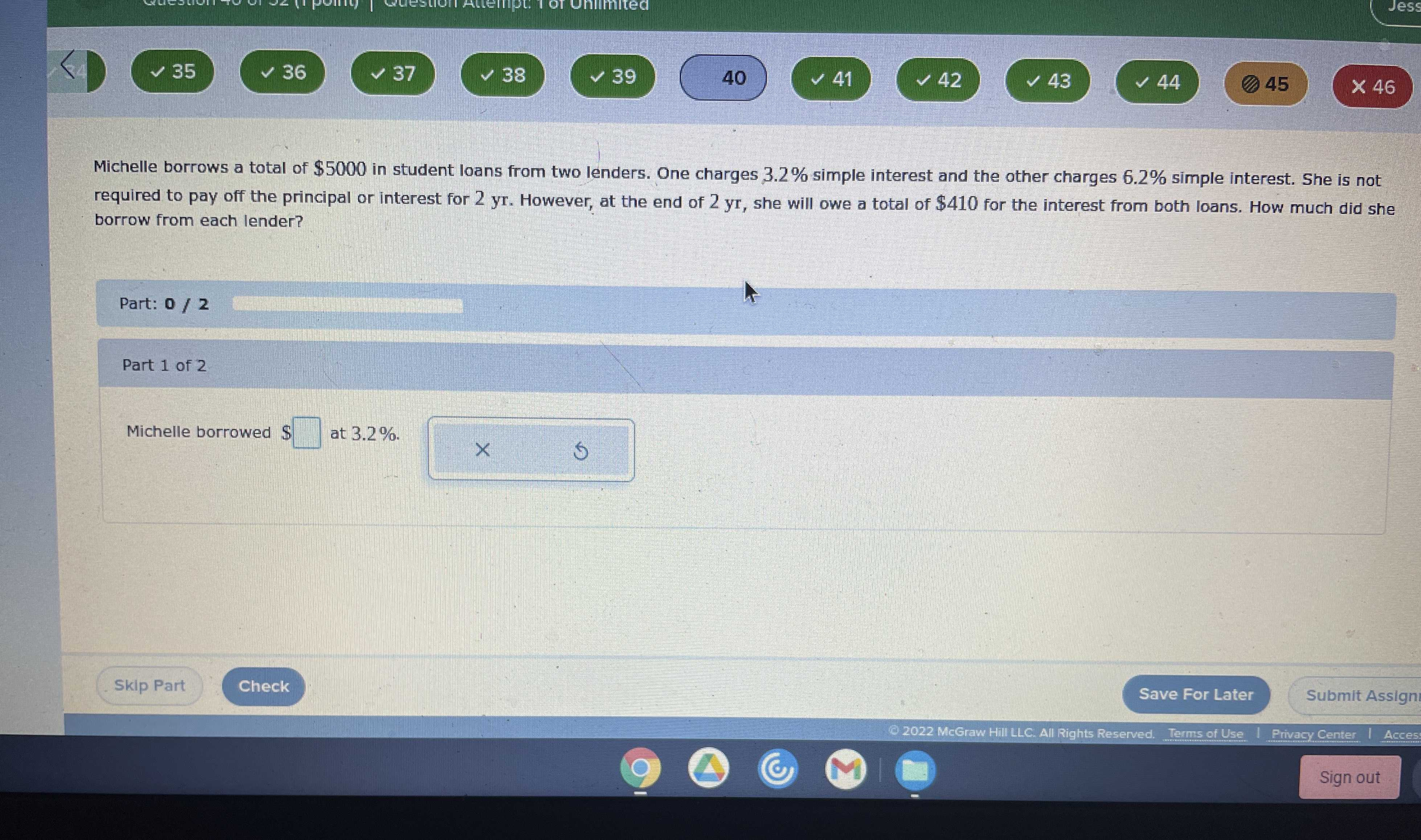### Still have math questions?

Algebra
QuestionMichelle borrows a total of $$\ 5000$$ in student loans from two lenders. One charges $$3.2 \%$$ simple interest and the other charges $$6.2 \%$$ simple interest. She is not required to pay off the principal or interest for $$2 yr$$ . However, at the end of $$2 yr$$ , she will owe a total of $$\ 410$$ for the interest from both loans. How much did she borrow from each lender?

Michelle borrowed $$\ \square$$ at $$3.2 \%$$